# Turán number

Jump to: navigation, search

A collection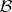of subsets of size( "blocks" ) of a ground set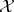of sizeis said to form a Turán-system if each-element subset ofcontains at least one block. The Turán number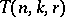is the minimum size of such a collection. P. Turán introduced these numbers in [a6]. The related dual notion is that of the covering number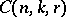, defined to be the smallest number of blocks needed to cover (by inclusion) each-element subset. Several recursions are known; e.g. in [a2] it is shown that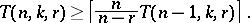Also, the limit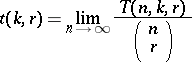is known to exist, though the values of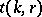are known only for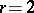. These facts and the ones that follow are based on an extensive survey by A. Sidorenko ([a5]):

i).

ii)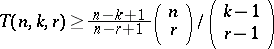[a1].

iii) It has been conjectured that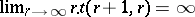[a1].

iv)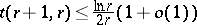[a4].

v)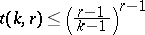[a3]. The situation of smallhas been studied extensively, as have been the cases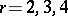. The case of small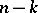is also well-studied; this leads to the covering number. See [a5] for details.